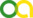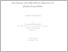(2021). Advanced data analysis for traction force microscopy and data-driven discovery of physical equations. PhD thesis, Universität zu Köln.Preview
PDF
Yunfei_Huang_thesis.pdf

## Abstract

The plummeting cost of collecting and storing data and the increasingly available computational power in the last decade have led to the emergence of new data analysis approaches in various scientific fields. Frequently, the new statistical methodology is employed for analyzing data involving incomplete or unknown information. In this thesis, new statistical approaches are developed for improving the accuracy of traction force microscopy (TFM) and data-driven discovery of physical equations. TFM is a versatile method for the reconstruction of a spatial image of the traction forces exerted by cells on elastic gel substrates. The traction force field is calculated from a linear mechanical model connecting the measured substrate displacements with the sought-for cell-generated stresses in real or Fourier space, which is an inverse and ill-posed problem. This inverse problem is commonly solved making use of regularization methods. Here, we systematically test the performance of new regularization methods and Bayesian inference for quantifying the parameter uncertainty in TFM. We compare two classical schemes, L1- and L2-regularization with three previously untested schemes, namely Elastic Net regularization, Proximal Gradient Lasso, and Proximal Gradient Elastic Net. We find that Elastic Net regularization, which combines L1 and L2 regularization, outperforms all other methods with regard to accuracy of traction reconstruction. Next, we develop two methods, Bayesian L2 regularization and Advanced Bayesian L2 regularization, for automatic, optimal L2 regularization. We further combine the Bayesian L2 regularization with the computational speed of Fast Fourier Transform algorithms to develop a fully automated method for noise reduction and robust, standardized traction-force reconstruction that we call Bayesian Fourier transform traction cytometry (BFTTC). This method is made freely available as a software package with graphical user-interface for intuitive usage. Using synthetic data and experimental data, we show that these Bayesian methods enable robust reconstruction of traction without requiring a difficult selection of regularization parameters specifically for each data set. Next, we employ our methodology developed for the solution of inverse problems for automated, data-driven discovery of ordinary differential equations (ODEs), partial differential equations (PDEs), and stochastic differential equations (SDEs). To find the equations governing a measured time-dependent process, we construct dictionaries of non-linear candidate equations. These candidate equations are evaluated using the measured data. With this approach, one can construct a likelihood function for the candidate equations. Optimization yields a linear, inverse problem which is to be solved under a sparsity constraint. We combine Bayesian compressive sensing using Laplace priors with automated thresholding to develop a new approach, namely automatic threshold sparse Bayesian learning (ATSBL). ATSBL is a robust method to identify ODEs, PDEs, and SDEs involving Gaussian noise, which is also referred to as type I noise. We extensively test the method with synthetic datasets describing physical processes. For SDEs, we combine data-driven inference using ATSBL with a novel entropy-based heuristic for discarding data points with high uncertainty. Finally, we develop an automatic iterative sampling optimization technique akin to Umbrella sampling. Therewith, we demonstrate that data-driven inference of SDEs can be substantially improved through feedback during the inference process if the stochastic process under investigation can be manipulated either experimentally or in simulations.

Item Type: Thesis (PhD thesis)
Creators:
CreatorsEmailORCIDORCID Put Code
Huang, Yunfeihuangyunfei586@gmail.comorcid.org/0000-0001-6519-3171UNSPECIFIED
URN: urn:nbn:de:hbz:38-356775
Date: 19 February 2021
Language: English
Faculty: Faculty of Mathematics and Natural Sciences
Divisions: Faculty of Mathematics and Natural Sciences > Department of Physics > Institute for Theoretical Physics
Subjects: Physics
Uncontrolled Keywords:
KeywordsLanguage
Traction force microscopyEnglish
Physical equationsEnglish
BiophysicsEnglish
Statistical physicsEnglish
Date of oral exam: 30 November 2020
Referee:
Gompper, GerhardProf. Dr.
Witthaut, DirkProf. Dr.
Refereed: Yes
URI: http://kups.ub.uni-koeln.de/id/eprint/35677View Item